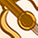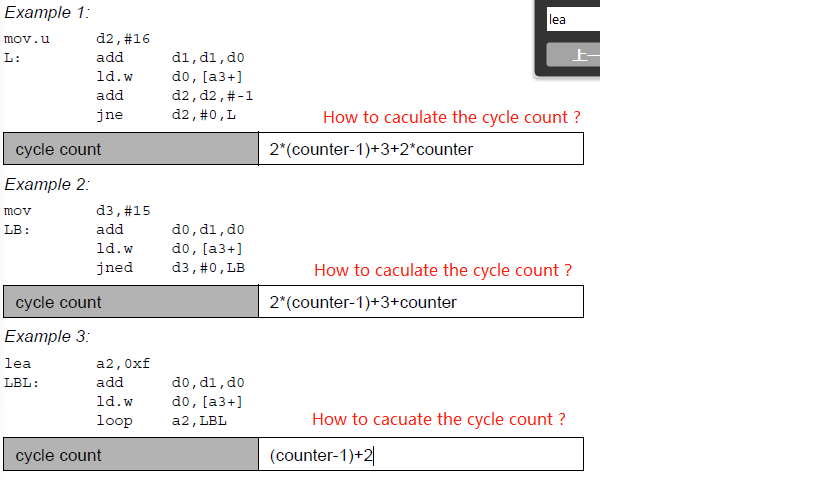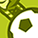Announcements

# AURIX™ Forum DiscussionsLevel 2
Level 2

# How to caculate the cycle count?

Hello, every one.
I found some problem after reading the "TriCore DSP Optimization Guide Part 1", in Page169:For Example, the "Example 1"
mov.u d2,#16
ld.w d0,[a3+]
jne d2,#0,L

"mov.u" is 1 cycle
"add d1,d1,d0" and "ld.w d0,[a3+]" is parralle, so is 1 cycle
jne d2, #0, L is 1 cycle
So, the total is: 1 + 4 * counter
but the document said:2*(counter-1)+3+2*counter
why?how to figure it out?

Another problem:
"ld.w d0,[a3+]", how to caculate the cycle of [a3+]?

2 RepliesLevel 2
Level 2

# Re: How to caculate the cycle count?

Is there anyone would like to tell me?Level 5
Level 5# Re: How to caculate the cycle count?

Hi Shaquille,

Here's the table I used:

`[IP] L: add.w     [LS]    ld.w            /* chapter 13.1.2 */[IP]    add       [BR]    jne       [2 or 3] /* chapter 13.6.1 */`

The ld.w instruction makes for a dual issue instruction. So combined with add.w it counts 1 single cycle. The jne instruction is two cycles when jumping backwards, and three cycles when evaluating to false. I left out the mov.u instruction because I think the focus was on the branch and not the preliminaries. This then results in the following calculous:

`cycles = (count-1)*(2+2) + 1*(2+3)       = 2*(count-1) + 2*(count-1) + 2 + 3       = 2*(count-1) + 2*count + 3`

So using words, for n iterations there are (n-1) of taken branches. And there is always 1 branch that will not be taken. The former is 4 cycles, the latter is 5 cycles. If someone can confirm or refute, that would be helpfull.

Best regards,

Henk-Piet Glas

Principal Technical Specialist
Embedded SoftwareThis widget could not be displayed.